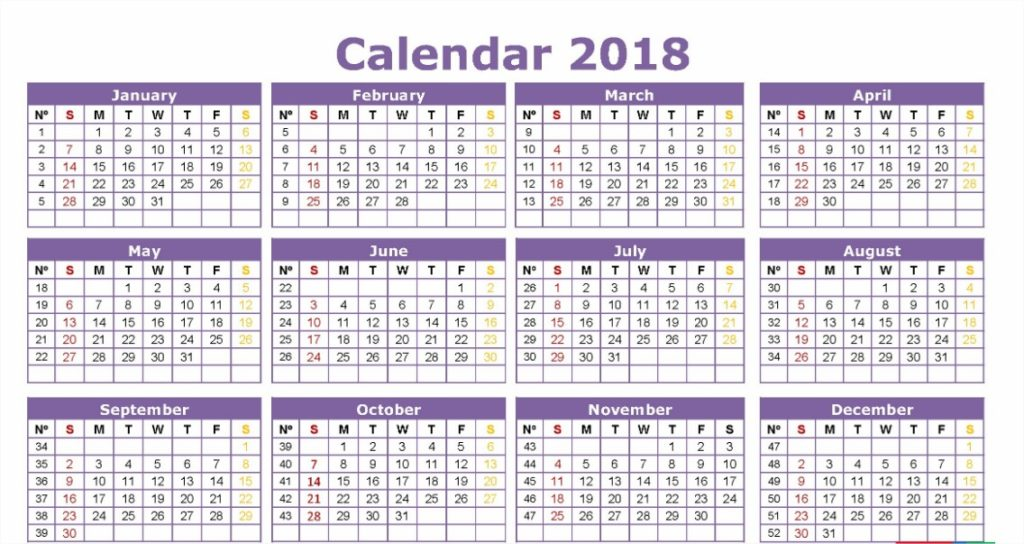# C Program To Print Number Of Days In A Month | 5 Ways

C program To Print Number Of Days In A Month – In this particular article, we will discuss the methods to print the number of days after inputting the month number.

Suitable examples and sample programs have also been added so that you can understand the whole thing very clearly. The compiler has also been added with which you can execute it yourself.

The ways used in this piece are as follows:

• Using Standard Method
• Using User-Defined Function
• Using Pointers

As we all know, there are 12 months in a regular calendar that is used on a day to day basis. This is also regarded as the Roman Calendar.

The latest month that was added was July, in the honour of Julius Caesar.As you can see, out of the total of 12 months:

• 7 months consist of 31 days
• 4 months consist of 30 days
• 1 month consists of 28 days (29 days if leap year)

Thus, the order of the months is the same all the time. Hence, by knowing the month number, we can decipher the number of days that month has.

Hence, the multiple ways to find out which month has how many days as per the month number in C programming is as follows:

## Using Standard Method

Output:

### Using User-Defined Function

1)Read the entered alphabet and store it in the variable m and pass the m to the user-defined function daysinmonths(m).

2)If the month number is within the range of 1 to 12,

then daysinmonths (int m)  checks whether the month number is equal to 2 or not,

if it is equal to 2 then it prints no.of days in  February. If it is not equal to 2 then it prints no.of days in the given month.

If it is not equal to 2 then it prints no.of days in the given month.

Output:

#### Using Pointers

1)Pass the address of array, address of entered alphabet to the function daysinmonths(Int *a, int *m).

2)The method daysinmonths() prints “Invalid input” if the value at the address of m is >12 or less than 1.

It prints “no.of days in month 2 is either 28 or 29” if the value at the address of m is 2.

If the value at the address of m is not >12 and not less than 1 and not equal to 2 then it prints no.of days in the given month.

Output:
x

## C Program To Print Number Of Days In A Month | Java Tutoring

C program to input the month number and print the number of days in that ...